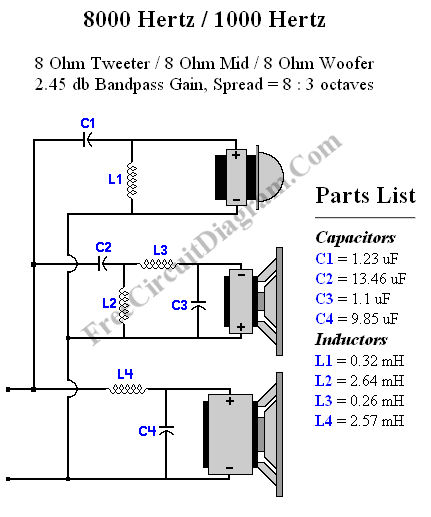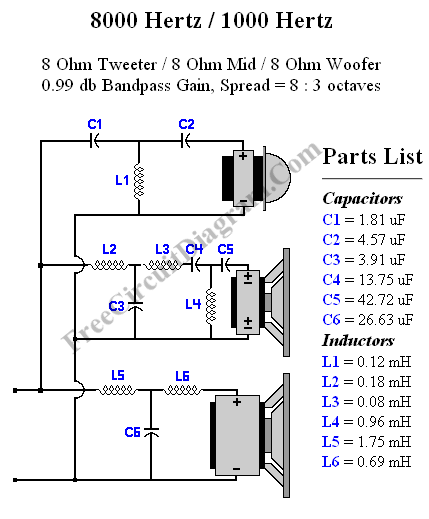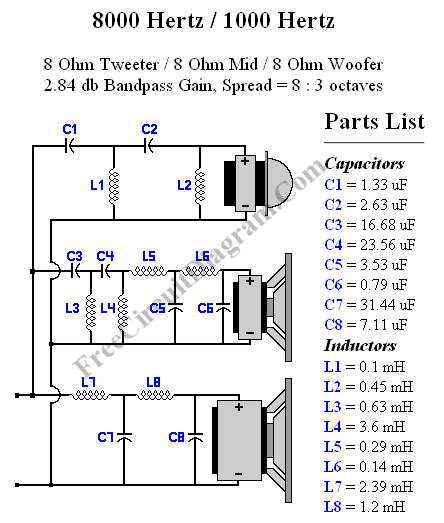# Crossover Design Using Online Crossover CalculatorCrossover Calculator

An online crossover calculator in this link is not only capable of designing 1st order crossover, but 2nd, 3rd, and 4th crossover calculation is also supported. Designing a 3 way crossover using the tool is very easy, you just need to fill the form to specify the design parameters: tweeter impedance, midrange impedance, and woover impedance, high crossover frequency, low crossover frequency, frequency spread, and the type or the order of the crossover design. Here are some examples of crossover design cases solved using the online crossover calculator:Figure 1. Second Order 3 Way Crossover Design Solved Using Crossover CalculatorFigure 2. Third Order 3 Way Crossover Design Solved Using Crossover CalculatorFigure 3. Fourth Order 3 Way Crossover Design Solved Using Crossover Calculator

You can see that the crossover calculator  shows you not only the components values, but also the schematic diagram of the crossover design. [Source: Crossover Calculator ]